# LU Decomposition With Pivoting

[Source: Lecture 21 in Trefethen-Bau Numerical Linear Algebra]Interchange row 1 and row 3 [left multiplication by P1]: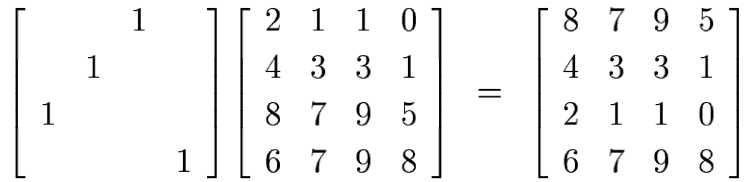Do elimination on the first column [multiplication by L1]: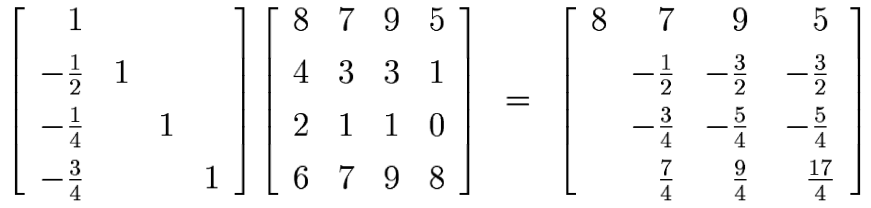Interchange rows two and four [multiplication by P2]: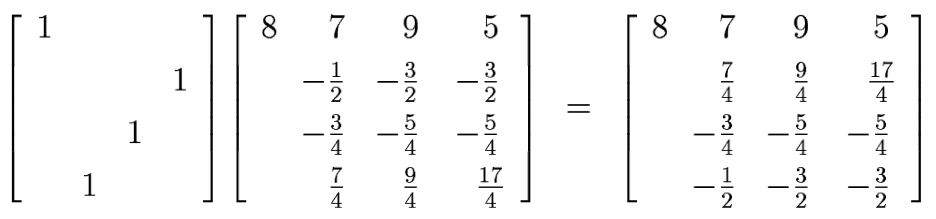Elimination on the second column [multiplication by L2]:Interchange rows three and four [multiplication by P3]: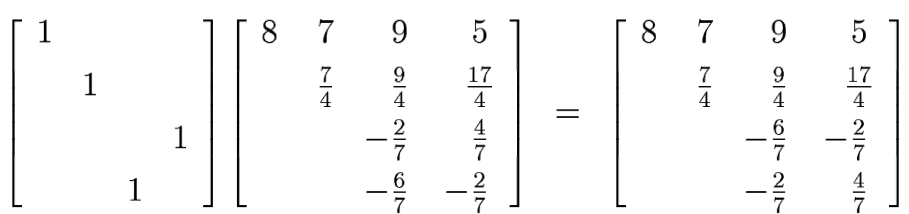Elimination on the third column [multiplication by L3]: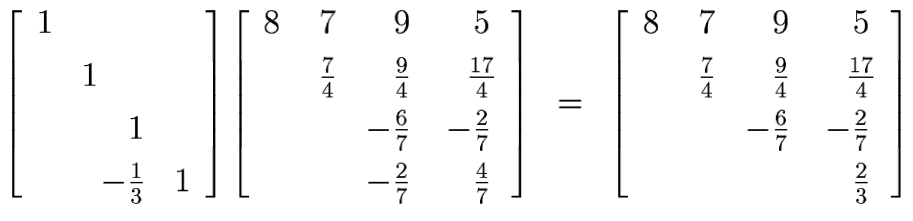Collecting the pieces we have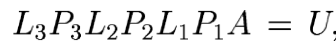where U is the upper triangular matrix on the right just above -- but the factors to the left of A are not at all lower triangular. We are lucky there is a fairly simple way out. These six elementary operations can be reordered in the form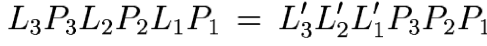(*)

where L'k is equal to Lk but with the subdiagonal entries permuted. To be precise, define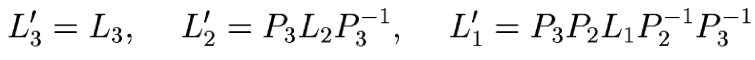Since each of these definitions apply only permutations Pj with j>k to Lk, one can verify that L'k has the same structure as Lk. Computing the product of the matrices L'k reveals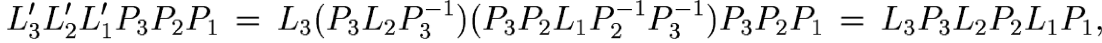as in equation (*).

The product of the matrices L'k is also unit lower triangular -- and also easily invertible by negating the subdiagonal entries., just as in Gaussian elimination without pivoting. Writing

L:=(L'3L'2L'1)-1   and   P= P3P2P1

we have the desired LU factorization of A
PA=LU
This has a pleasant interpretation:
1. Permute the rows of A using P.

2. Apply Gassian elimination without pivoting to PA.
In theory, this gives insight. But in practice it doesn't help since we don't know P until the end of the computation.

Note that scaling the rows of A to choose the pivot point does not change this since the choice of the pivot point is a separate issue.